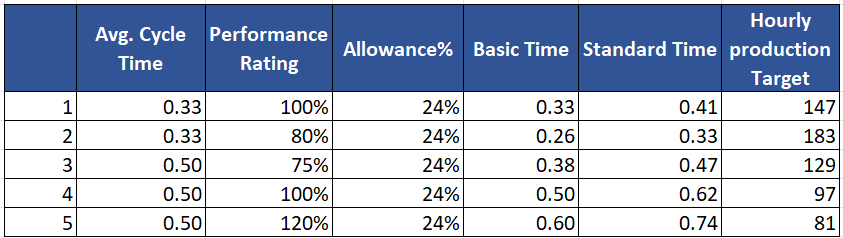# Why is Cycle Time Multiplied by Rating to Calculate Standard Minutes?

### Question and Confusion:

The average cycle time of an operation is 0.33 minutes, but one analyst rates the operator at 100% and another analyst rates the same operator at 80%.
As for the assignments, a 24% is granted.
Considering that the difference is only in the rating given by each analyst, the standard time in the first case is (0.33 × 1 × 1.24)= 0.41 Minutes. In the second case, it is (0.33 × 0.80 × 1.24) = 0.33 minutes

The question is why in case 1, where the operator is better rated, the time is greater than in case 2 where the operator is rated with a lower percentage?

This is related to the production capacity per hour, while in case 1 there are 146 units (60÷0.41) and in case 2 there are 181 units (60÷0.33). Shouldn’t it be that with a rating of 100% the production capacity be higher than with an 80% rating. Can you explain the mathematical logic of this case to me?

### Answer to the first question:

The standard time calculation method from the average cycle time shown above is correct. Also, the hourly production capacity of the operators is correct in the above query.

One needs to understand the definition of Standard Time. It is the time a 100% performing operator would take to complete an operation.

Standard Time = (Cycle Time X Rating%) + Allowance%
Basic time =  (Cycle Time X Rating%)

For the time being keep the allowance of 24% aside. We will compare the calculated basic time after multiplying the average cycle time by the rating. We will get the standard time after adding various allowances to the Basic Time.

In the second case, the average cycle time is 0.33 minutes, and with an 80% rating, you are getting Basic time = (0.33 X 0.80) = 0.264 minutes.

The calculated Basic Time (0.264 minutes) is less than the observed time (0.33 minutes) and it is correct. This matches with the reality that the operator with a higher rating (>80%) would take less time (<0.33 minutes).

According to the analyst, the operator is taking more time than standard time which is why he has rated her as 80%.

Now, add the 24% allowance to both cases and see the calculated Standard Time.

I hope you understand my explanation of why the average cycle time is multiplied by the rating factor.  Here are a few examples of conversion from cycle time to standard time.https://www.onlineclothingstudy.com/2014/11/how-to-use-performance-rating.html

### Capacity Calculation

The calculation of hourly capacity (based on standard time) in the above query is correct.
Hourly production capacity = (60 minutes / Standard Time)

Instead of 'production capacity', you can call it potential production capacity or Hourly production target at 100% Standard Time.

The correct way of calculating hourly capacity is (60 minutes / Average cycle time)+Allowance.

This mathematical calculation will give a better projection of hourly capacity. Reason - operators will work as per their skill level and most of the time operators do not perform as per standard time. So, there will be a gap between the standard time and cycle time of an operation.